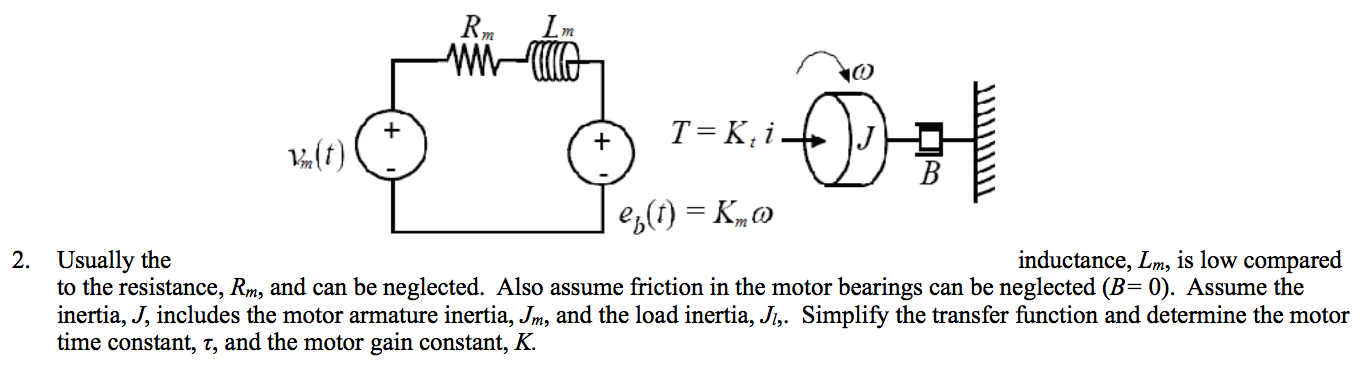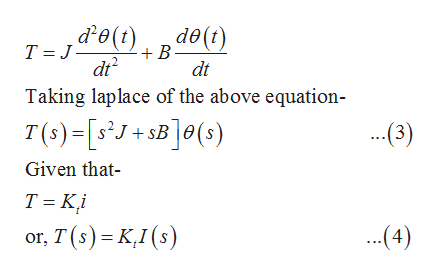# mT K, i-Bep)Kinductance, Lm, is low comparedUsually theto the resistance, Rm, and can be neglected. Also assume friction in the motor bearings can be neglected (B= 0). Assume theinertia, J, includes the motor armature inertia, Jm, and the load inertia, Ji,. Simplify the transfer function and determine the motortime constant, 7, and the motor gain constant, K.2.

Questionhelp_outlineImage Transcriptionclosem T K, i- B ep)K inductance, Lm, is low compared Usually the to the resistance, Rm, and can be neglected. Also assume friction in the motor bearings can be neglected (B= 0). Assume the inertia, J, includes the motor armature inertia, Jm, and the load inertia, Ji,. Simplify the transfer function and determine the motor time constant, 7, and the motor gain constant, K. 2. fullscreen
check_circle

Step 1

The transfer function is the ratio of the Laplace transform of output to the input into the system.

In a series motor, the terminal voltage is the input and the shaft displacement is the output.

Solving the electrical circuit in the Laplace domain-

Applying KVL-

Step 2

At the shaft-help_outlineImage Transcriptionclose,&O(t) d()de(t) T J B dt dt Taking laplace of the above equation- T (s)-[sJ+sB]0(s) ..(3) Given that T Ki (4) or, T(s) K,I (s) fullscreen
Step 3

From equation (3)...

### Want to see the full answer?

See Solution

#### Want to see this answer and more?

Solutions are written by subject experts who are available 24/7. Questions are typically answered within 1 hour.*

See Solution
*Response times may vary by subject and question.
Tagged in

### Electrical Engineering